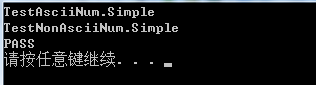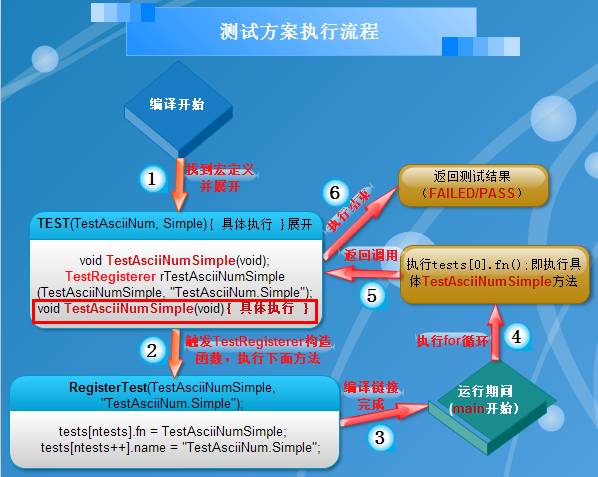# 第一步：写个头文件，定义测试所用类和测试方法。

// test.h#define TEST(x, y) \
void x##y(void); \
TestRegisterer r##x##y(x##y, # x "." # y); \
void x##y(void)

void RegisterTest(void (*)(void), const char*);

class TestRegisterer {
public:
TestRegisterer(void (*fn)(void), const char *s) {
RegisterTest(fn, s);
}
};

1. 一个函数指针：void (*fn)(void)，指向我们具体要编写的测试方法名；

2. 一个字符串：const char *s，属于该测试方法的描述信息。

TEST(x, y) {
....      // 具体实现
}

void testflow(void);
TestRegisterer rtestflow(testflow, "test.flow");
void testflow(void) {
....      // 具体实现
}

# 第二步：N多个具体的测试实现。

#include <string>
#include <vector>
#include "test.h"

#define arraysize(array) (sizeof(array)/sizeof((array)))
#define CHECK_EQ(x, y)    if((x) != (y)) { printf("test failed!\n"); system("pause"); exit(0); }

struct TestFlow {
const char* flow;
const int num;
};

static struct TestFlow tests1[] = {
{"\x02\x97\xa4\xe6\xfe\x0c", 2},
{"\x05\x97\x35\xe6\xfe\xac\x04", 3},
{"\xb2\x97\xa5\xe6\x9c\x1c\x58\xaa\x97\x03", 3},
{"\x32\x97\xa5\x05\x9c\xac\xe8\xaa\x57", 3},
{"\x42\x01\xa5\x86\x0c\x56\xe8\xaa\x97\x03", 5},
};

static struct TestFlow tests2[] = {
{"\x02\x97\xa4\xe6\xfe\x0c", 4},
{"\x05\x97\x35\xe6\xfe\xac\x04", 4},
{"\xb2\x97\xa5\xe6\x9c\x1c\x58\xaa\x97\x03", 7},
{"\x32\x97\xa5\x05\x9c\xac\xe8\xaa\x57", 6},
{"\x42\x01\xa5\x86\x0c\x56\xe8\xaa\x97\x03", 5},
};

int getAsciiNum(const char*);
int getNonAsciiNum(const char*);

TEST(TestAsciiNum, Simple) {
int failed = 0;
for (int i = 0; i < arraysize(tests1); i++) {
const TestFlow& t = tests1[i];
int num = getAsciiNum(t.flow);
if (num != t.num) {
failed++;
}
}
CHECK_EQ(failed, 0);
}

TEST(TestNonAsciiNum, Simple) {
int failed = 0;
for (int i = 0; i < arraysize(tests2); i++) {
const TestFlow& t = tests2[i];
int num = getNonAsciiNum(t.flow);
if (num != t.num) {
failed++;
}
}
CHECK_EQ(failed, 0);
}

int getAsciiNum(const char* flow) {
// we assume that there's no \x00 in flow otherwise we cannot use strlen()
int num = 0, i;

for(i = 0; i < strlen(flow); i++) {
// ASCII: 0 ~ 127
if(flow[i] >= 0 && flow[i] < 128)
num++;
}

return num;
}

int getNonAsciiNum(const char* flow) {
// we assume that there's no \x00 in flow otherwise we cannot use strlen()
int num = 0, i;
for(i = 0; i < strlen(flow); i++) {
// ASCII: 0 ~ 127
if(flow[i] < 0 || flow[i] >= 128)
num++;
}

return num;
}

# 第三步：具体的测试方案。

// test.cpp
#include <stdio.h>
#include <stdlib.h>
#include "test.h"

struct Test {
void (*fn)(void);
const char *name;
};

static Test tests;
static int ntests;

void RegisterTest(void (*fn)(void), const char *name) {
tests[ntests].fn = fn;
tests[ntests++].name = name;
}

int main(int argc, char **argv) {
for (int i = 0; i < ntests; i++) {
printf("%s\n", tests[i].name);
tests[i].fn();
}
printf("PASS\n");
system("pause");

return 0;
}

1. 结构体Test存储具体的测试实现，定义最多能有10000个不同的方法测试，也就是能同时测试10000个方法。

2. ntests代表实际所测试的方法数，我这里就是2了。

3. RegisterTest()具体的实现也比较简单，就是将实际所要测试的方法名和描述信息存储到Test结构体数组tests中。

4. 最后就是在main中进行统一测试了，首先输出测试方法描述信息，以便知道当前测试了哪些方法及如果有测试失败时能及时进行排查。然后就是具体的执行测试函数了。# 思考：下面看下具体是如何执行的：

TEST(TestAsciiNum, Simple)展开为：

void TestAsciiNumSimple(void);
TestRegisterer rTestAsciiNumSimple(TestAsciiNumSimple, "TestAsciiNum.Simple");
void TestAsciiNumSimple(void) {
int failed = 0;
for (int i = 0; i < arraysize(tests1); i++) {
const TestFlow& t = tests1[i];
int num = getAsciiNum(t.flow);
if (num != t.num) {
failed++;
}
}
CHECK_EQ(failed, 0);
}posted @ 2013-11-16 14:00  Alexia(minmin)  阅读(7758)  评论(4编辑  收藏